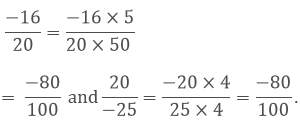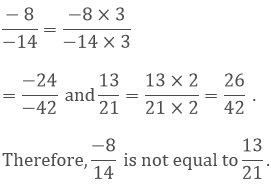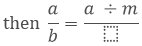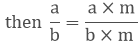×#### Thank you for registering.

One of our academic counsellors will contact you within 1 working day.

Click to Chat

1800-1023-196

+91-120-4616500

CART 0

• 0

MY CART (5)

Use Coupon: CART20 and get 20% off on all online Study Material

ITEM
DETAILS
MRP
DISCOUNT
FINAL PRICE
Total Price: Rs.

There are no items in this cart.
Continue Shopping• Complete JEE Main/Advanced Course and Test Series
• OFFERED PRICE: Rs. 15,900
• View Details

```Chapter 4: Rational Numbers Exercise – 4.5

Question: 1

Which of the following numbers are equal?

(i) – 9/12 and 8/-12

(ii)  -16/20 and 20/-25

(iii)  -7/21 and 3/-9

(iv)  – 8/-14 and 13/21

Solution:

(i) The standard form of -9/12 is -9/3, 12/3 = -34

The standard form of 8/-12 is 8/-4, 12/-4 = -2/3

Since, the standard forms of two rational numbers are not same. Hence, they are not equal.

(ii) Since, LCM of 20 and 25 is 100.

Therefore making the denominators equal,Therefore, -16/20 = 20/-25 .

(iii) Since, LCM of 21 and 9 is 63.

Therefore making the denominators equal,(iv) Since, LCM of 14 and 21 is 42.

Therefore making the denominators equal,Question: 2

If each of the following pairs represents a pair of equivalent rational numbers,

find the values of x:

(i)  2/3 and 5/x

(ii) -3/7 and x/4

(iii)  3/5 and x/-25

(iv) 13/6 and - 65/x

Solution:

(i) 2/3 = 5/x, then x = (5 × 3)/2 = 15/2

(ii) -3/7 = x/4, then x = (-3/7) × 4 = -12/7

(iii)  3/5 = x/-25, then x = 3/5 x (-25) = -75/5 = -15

(iv) 13/6 = – 65/x , then x = 6/13 x (- 65) = 6 x (-5) = -30

Question: 3

In each of the following, fill in the blanks so as to make the statement true:

(i)  A number which can be expressed in the form p/q, where p and q are integers and q is not equal to zero , is called a ………..

(ii)  If the integers p and q have no common divisor other than 1 and q is positive, then the rational number p/q is said to be in the ….

(iii) Two rational numbers are said to be equal, if they have the same …. form

(iv) If m is a common divisor of a and b,(v)  If p and q are positive Integers, then p/q is a …..rational number and  p/(-q)  is a …… rational number .

(vi)  The standard form of -1 is …

(vii)  If p/q is a rational number, then q cannot be ….

(viii)  Two rational numbers with different numerators are equal, if their numerators are in the same …. as their denominators .

Solution:

(i)  rational number

(ii)  standard rational number

(iii)  standard form

(iv)  a/b = (a ÷ m)/(b ÷ m )

(v)  positive rational number, negative rational number

(vi) -1/1

(vii)  Zero

(viii)  ratio

Question: 4

In each of the following state if the statement is true (T) or false (F):

(i) The quotient of two integers is always an integer.

(ii) Every integer is a rational number.

(iii) Every rational number is an integer.

(iv) Every traction is a rational number.

(v)  Every rational number is a fraction.

(vi) If a/b is a rational number and m any integer,(vii)  Two rational numbers with different numerators cannot be equal.

(viii)  8 can be written as a rational number with any integer as denominator.

(ix) 8 can be written as a rational number with any integer as numerator.

(x)  2/3 is equal to 4/6.

Solution:

(i)  False; not necessary

(ii)  True; every integer can be expressed in the form of p/q, where q is not zero.

(iii)  False; not necessary

(iv) True; every fraction can be expressed in the form of p/q, where q is not zero.

(v) False; not necessary

(vi) True

(vii) False; they can be equal, when simplified further.

(viii) False

(ix) False

(x)  True; in the standard form, they are equal.
```### Course Features

• 728 Video Lectures
• Revision Notes
• Previous Year Papers
• Mind Map
• Study Planner
• NCERT Solutions
• Discussion Forum
• Test paper with Video Solution